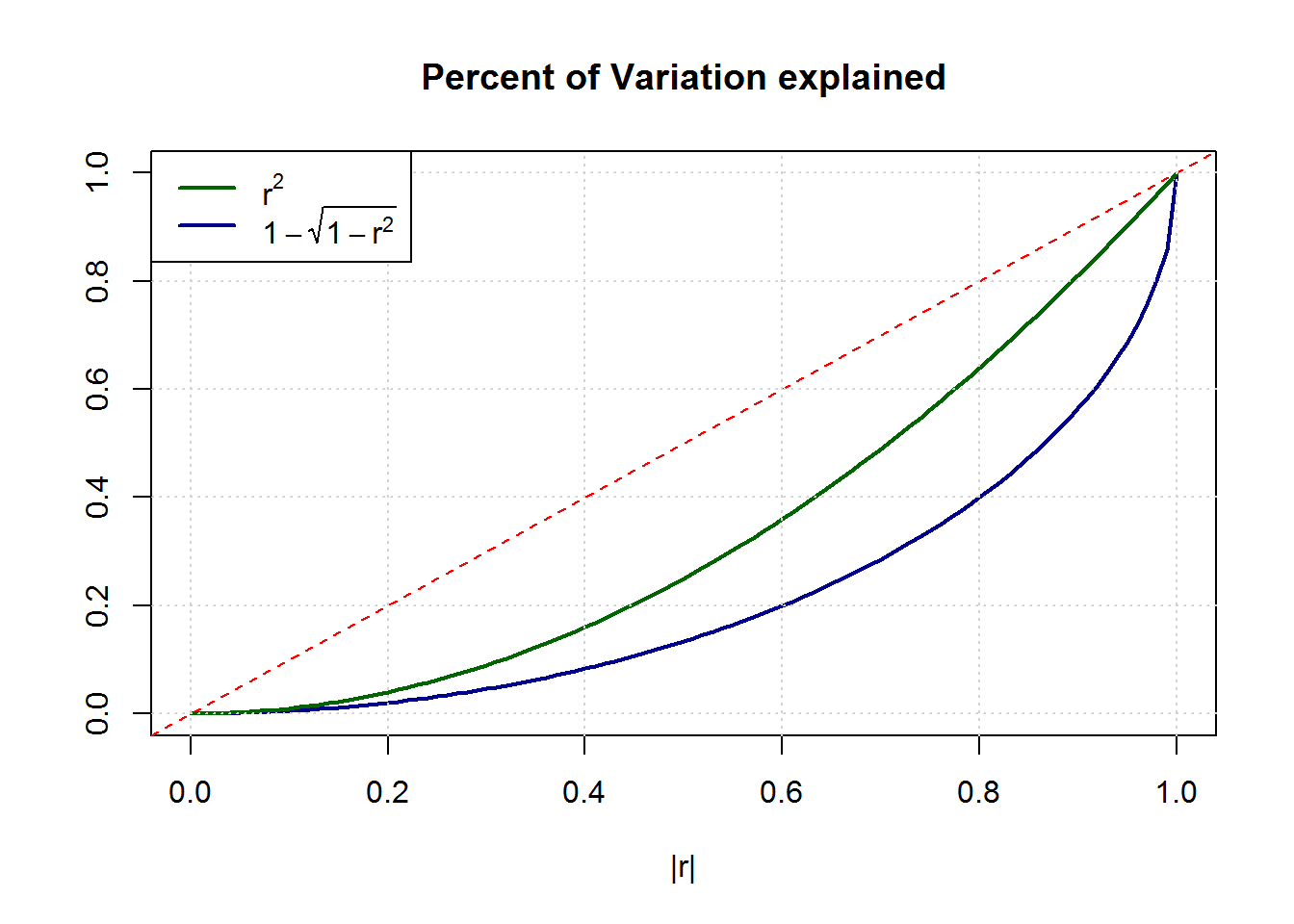# Coefficient of Determination

R^2 Statistic

It seems to odd to me that we measure the explanatory power of a regression model in “percent of variance explained”, or $$R^2 = cor(\hat{y},y)^2 = r^2$$ even though we all know that variance is just an auxiliary quantityto compute the more meaningful measure of uncertainty which is the standard deviation. Risk in finance or uncertainty in prediction is measured by $$\sigma$$, not by $$\sigma^2$$. Knowing the reduction in variance in a regression model seems much less useful than the reduction in stdev.

In fact, whenever I try to explain $$R^2$$ to my students, I usually start by comparing the overall variation of y (as measured by $$\sigma_y$$) to the remaining variation around the regression line (measured by $$\sigma_{\epsilon}$$). That idea is adapted much more naturally than the comparison of the variances which really have no direct interpretation!

So I propose a new measure which is truly “the amount of standard deviation explained”. We can quickly derive it: $R^2 (=r^2) = 1 – \frac{RSS}{TSS} \Leftrightarrow 1 – \frac{\sqrt{RSS}}{\sqrt{TSS}} = 1-\sqrt{1-r^2}$
where RSS = “residual sum of squares” ($$\approx \sigma_{\epsilon}^2$$) and TSS = “total sum of squares” ($$\approx \sigma_{y}^2$$)

Comparing the traditional $$r^2$$ with the new measure $$1-\sqrt{1-r^2}$$ reveals that substantially stronger correlations $$cor(\hat{y},y)$$ are needed to result in similar “uncertainty reduction”. E.g. what one used to call a high value of $$R^2 = 0.8$$ explaining 80% of the variance, would have reduced the true uncertainty by merely 55% !

The graph below shows the stronger convexity of this alternative measure.